# Mandala Fractal

Group Members

Collin Cullen (Math Consultant)
Dillon Call (Designer)

First Design

For our very first design, we were inspired by some mandala pictures that we found, and they resembled fractals because a mandala is just several levels of rings with different self-similar designs in each ring. We wanted to construct it in a way that it would look original, yet fulfill the criteria of the project and actually be a true fractal. We would like our fractal to be formed so that there would be a never ending pattern that repeats itself at different scales. As of right now we are still working on our design on Tinkercad, but we find it a bit challenging to make the iterations and to make sure that it is an actual fractal. Hopefully we can make a design that is not too complicated and easily printable. We have made our fractal so that each iteration is 4/5 the height and the circumference of the first level.

Here are some mandala pictures that inspired our fractal.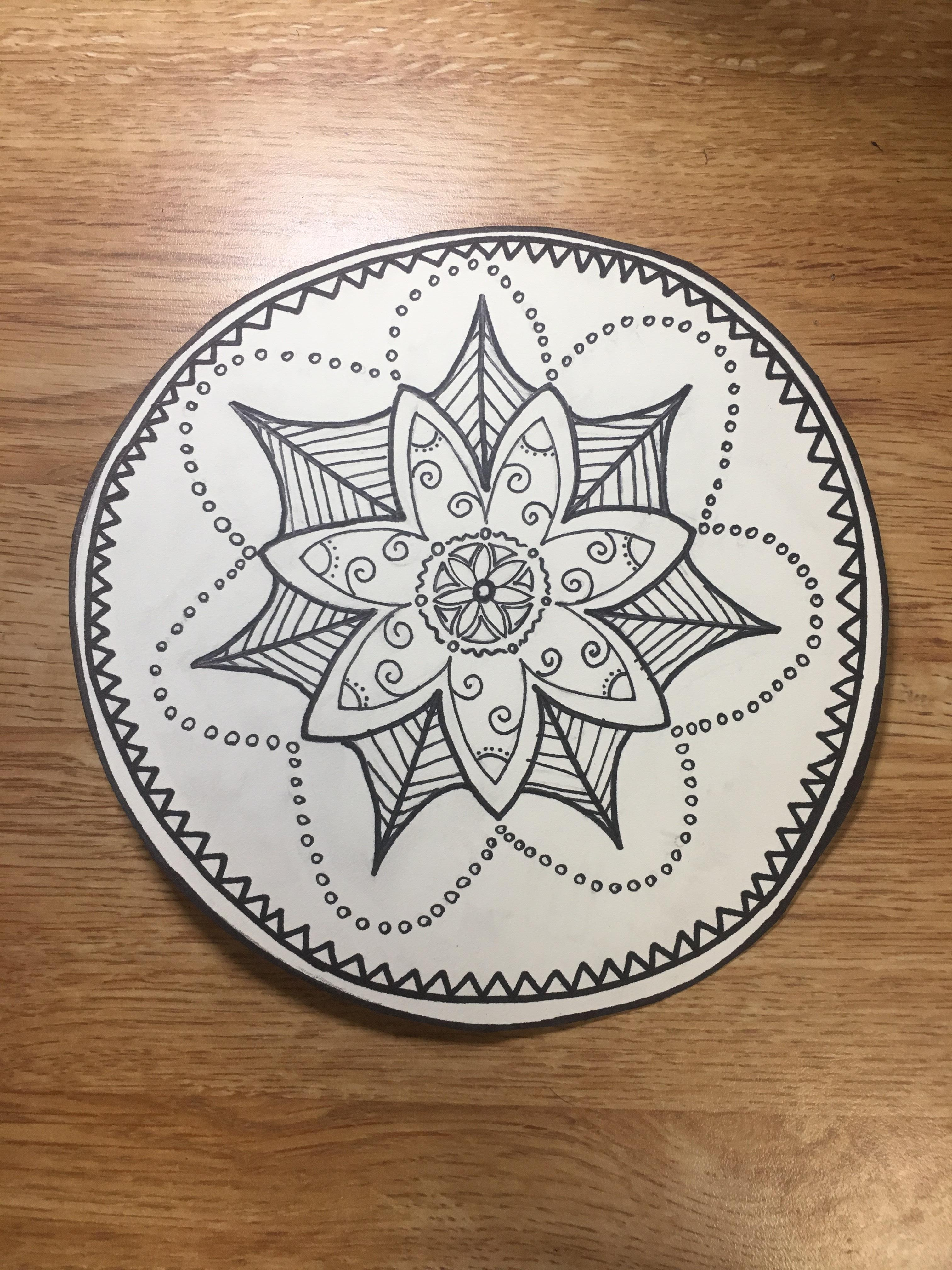Below is the original design: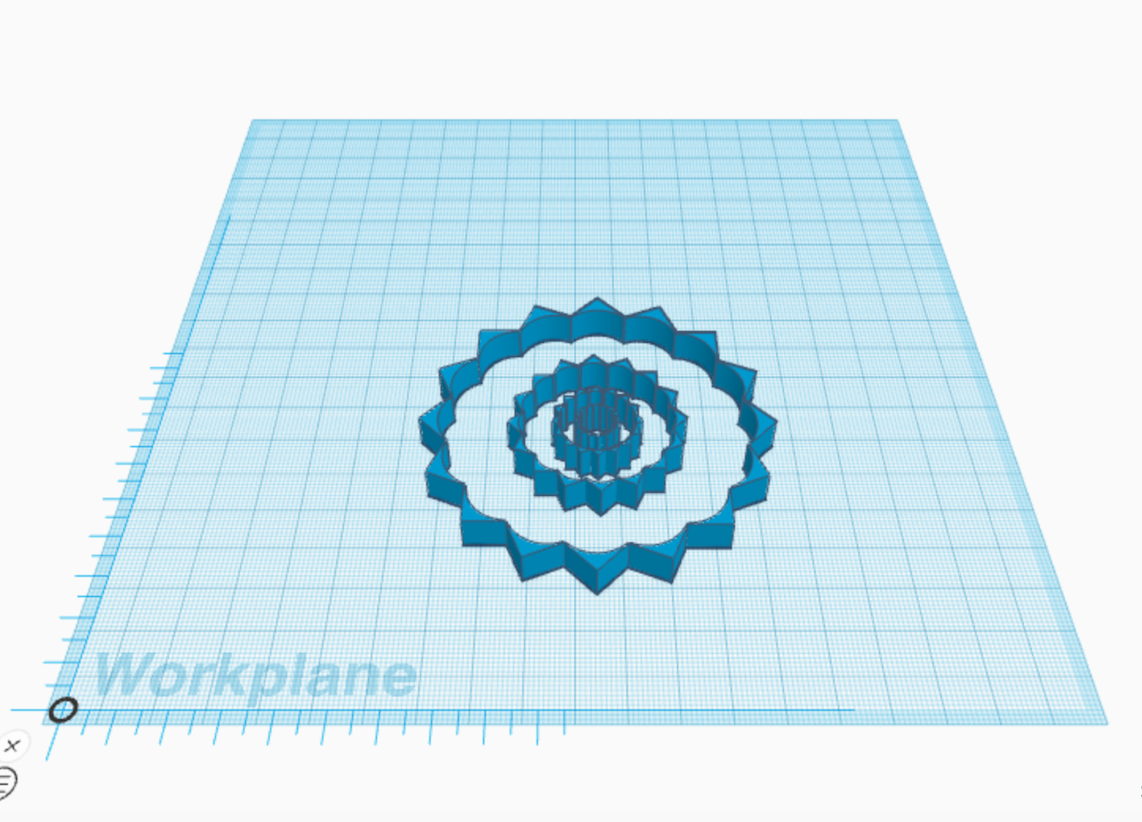Below is the final design that we sent to the Ultimaker to print. As you can see we added more layers so that the figure would look more packed. Initially we made the second level only 1/2 of the original one in terms of height and circumference. However for the final design we made the height and circumference to be 4/5 of the first level. We could think our fractal in a way in which the first level is the outermost layer and that with each new level and iteration the fractal gets smaller and smaller. If this were the case, we think that our fractal would go maybe go infinitely smaller. However, we also thought that perhaps at some point the fractal could crash with each other, since its getting smaller not only in terms of height and circumference, but also the radius. To print the complete fractal it would take us about four hours, but we managed to adjust the size so that it could print in about forty minutes. Hopefully we are able to see our beautiful design print nicely on the printers.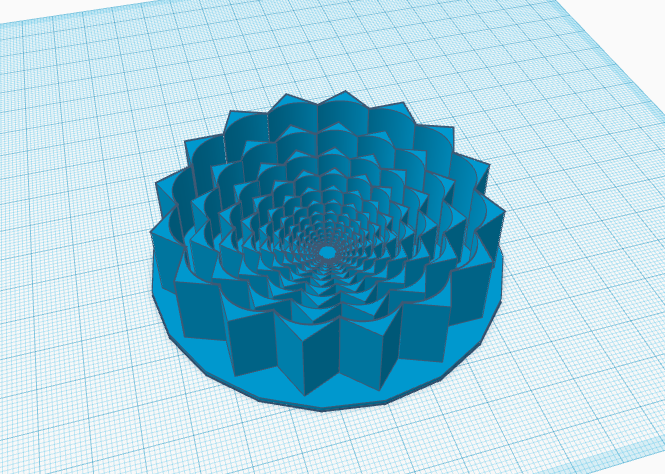3D Printed Result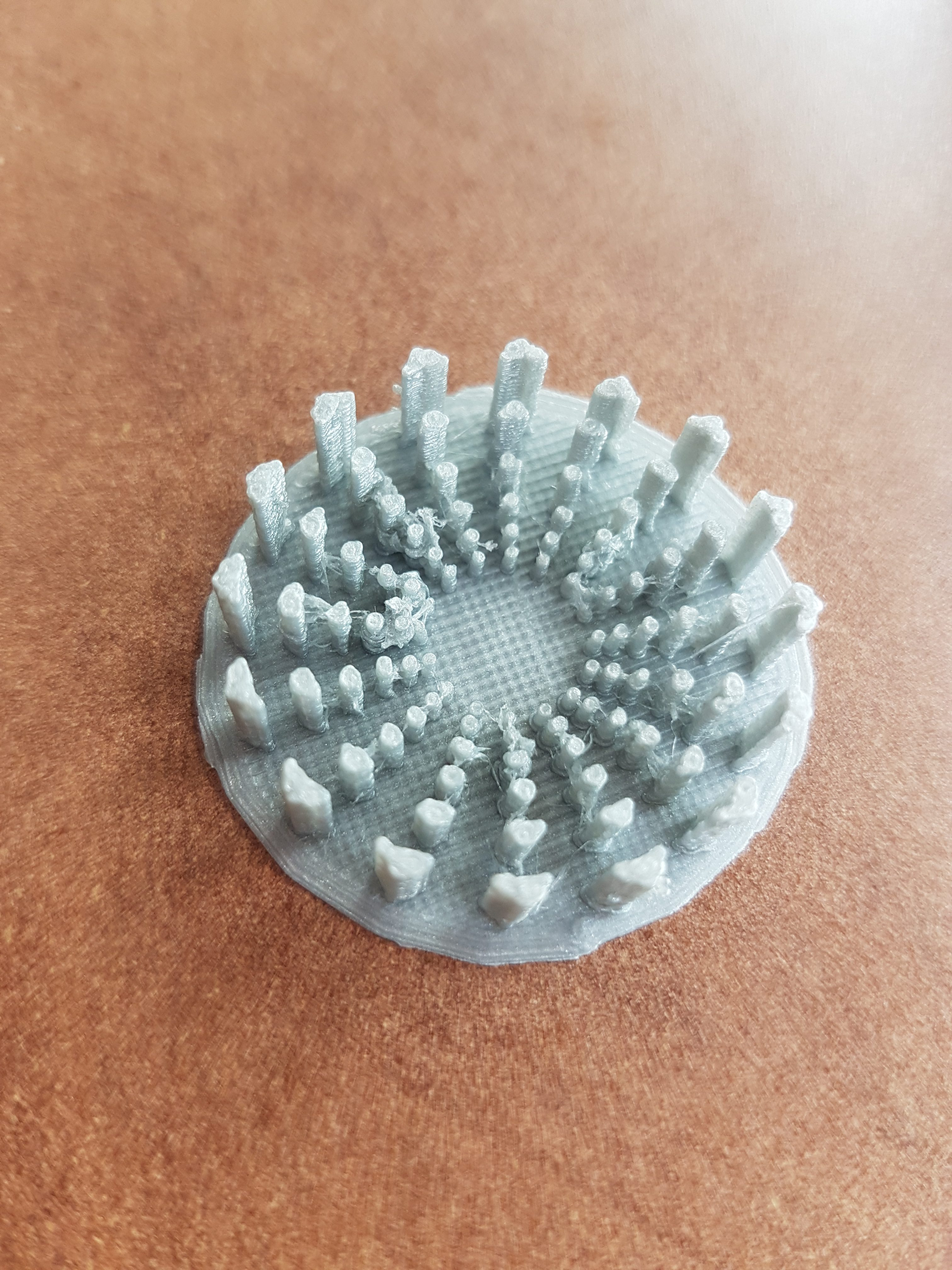The above photo was our first attempt. We had to shrink it down by 30% to get the print finished within our class period. It only took about 45 minutes compared to four hours for our final design. However, it isn’t as detailed, and it pretty much is just the innermost iterations of our final design.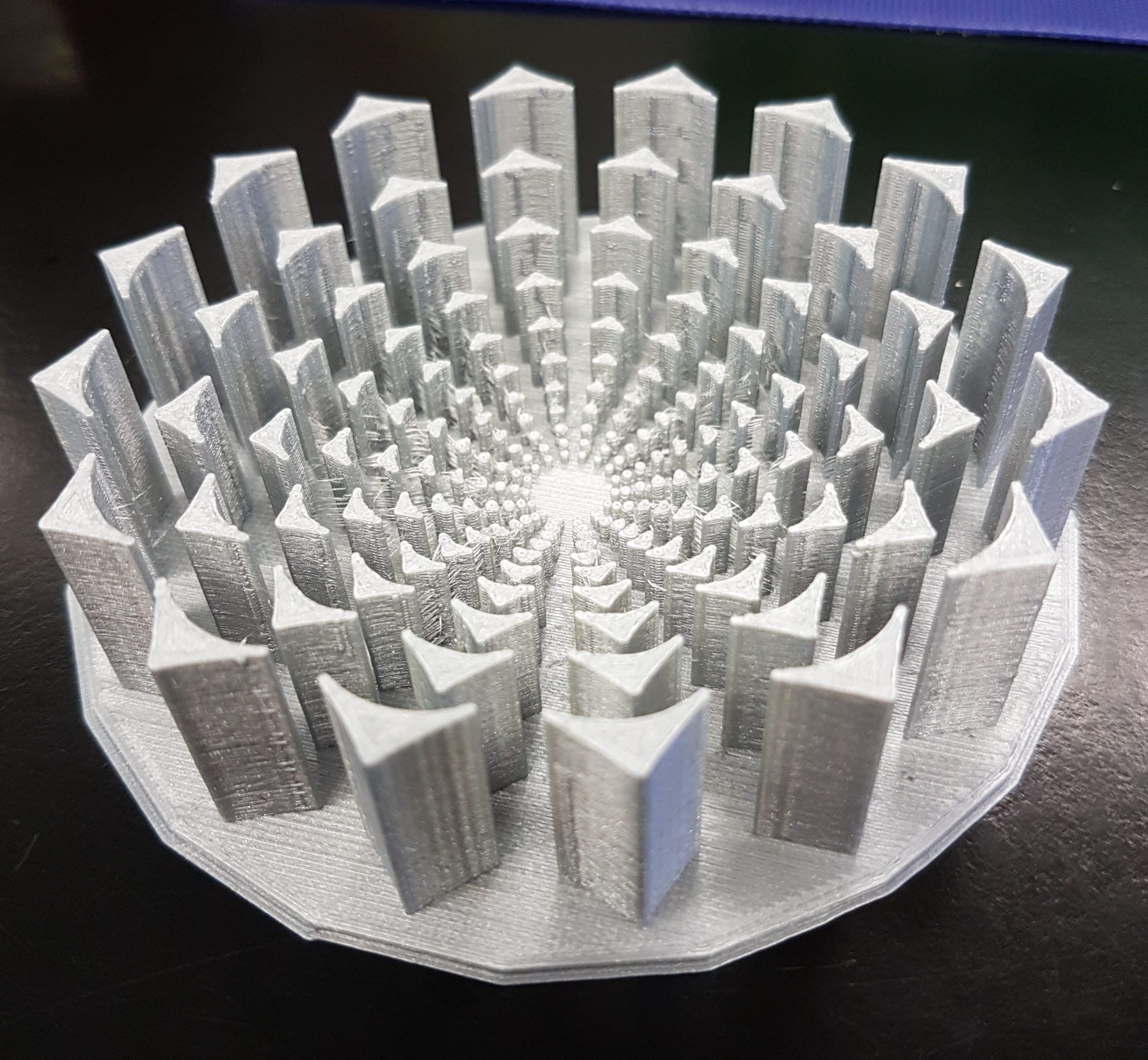Our print result looks great after coming out of the Ultimaker after the four hour process. However, one thing that we were concerned about is the fact that there is a bit of space between the triangular shapes, even though in the Tinkercad design there is no space at all. However, this would not be a big problem, since we are still able to measure and do some calculations on our printed fractal. The print was still successful in making each new iteration 4/5 of the previous one.

Volume Calculations

In order to calculate the volume of our fractal, we started out with the first level to have a volume of 1. We wanted it to be easier to calculate, and we wanted to make sure that we would be able to calculate each smaller iteration as accurately as possible. What we know is with each level, the fractal will be 4/5 times smaller than the original one in terms of length, volume and height. We figured out that our fractal would have an infinite volume since we kept adding the previous level/iteration together after each level that we created.

Level 0

V=1

Level 1

V1= V + ( .8^3)

V1= 1 + (.512)

V1= 1.512

Level 2

V2= V + V1 ( .8^3)

V2= 1 + 1((.8^3)^2)

V2= 1.262

Level 3

V3= V + V2 ((.8^3))
V3 = 1 + 1((.8^3)^2))
V3 = 1.134

Level 100

1+ 1( .8)^3 + 1 ( .8^3)( .8^3) + 1( .8^3)( .8^3)( .8^3) + … + 1(.8^3)^100

Level n

1+ 1( .8)^3 + 1 ( .8^3)( .8^3) + 1( .8^3)( .8^3)( .8^3) + … + 1(.8^3)^n

Fractal Dimension

We know that our fractal is scaled down by 4/5, and it divides into different subsets. Because we cannot calculate dimension at this time, we will be calculating the dimension of the Sierpinski triangle.

(1/2)^D = (1/3)
2^D=3
D= ” log2 (3)” = log 3/ log 2 = 1.58496

S^D = 1/N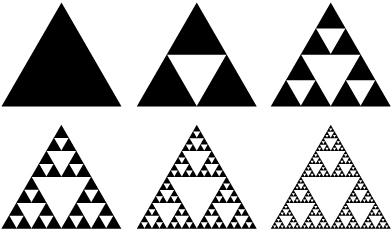How To Find Our Models:

Check our design on both tinkercad and thingiverse.

#### 2 Comments on Mandala Fractal

1. I think you only need to include the volume column, not area or height, because each level scales uniformly. Try doing levels 3 and 4 to see the pattern so you can do level 100 and level n. And don’t forget infinity

2. Can you include a picture of the Sierpinski triangle in the dimension section and refer to it to explain your calculations in that section?